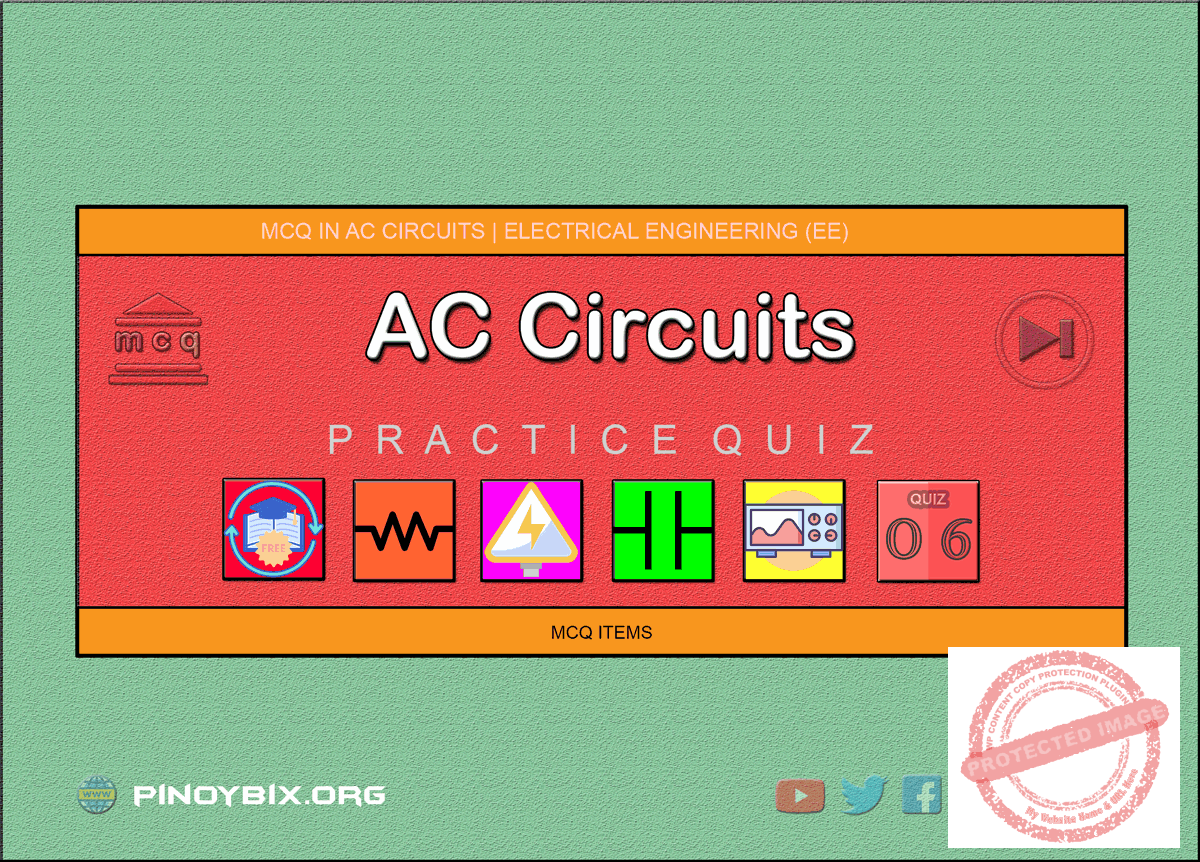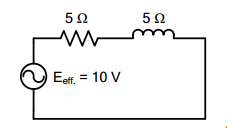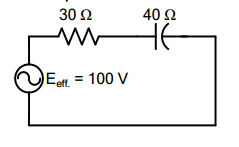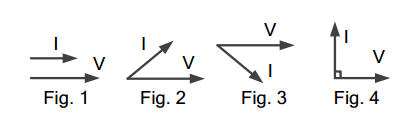# MCQ in AC Circuits Part 6 | REE Board Exam

(Last Updated On: November 13, 2020)This is the Multiple Choice Questions Part 6 of the Series in AC Circuits as one of the Electrical Engineering topic. In Preparation for the REE Board Exam make sure to expose yourself and familiarize in each and every questions compiled here taken from various sources including but not limited to past Board Exam Questions in Electrical Engineering field, Electrical Engineering Books, Journals and other Electrical Engineering References.

#### Continue Practice Exam Test Questions Part 6 of the Series

SERIES CIRCUITS

Choose the letter of the best answer in each questions.

251.  A circuit draws a current of (3 – j8) A from a source of (100 + j37) V. Find the true power of the circuit.

A.  4 W

B.  596 W

C.  300 W

D.  296 W

Solution:

252.  A  resistor  and  a  coil  are  connected  in  series  with  a  voltage  source.  If  the voltage  across  the  coil  is  10  sin  (866t  +  70°)  V  and  the  current  flowing through the resistor is 2 cos (866t – 80°) A, what is the resistance of the coil?

A.  4.92 Ω

B.  2.5 Ω

C.  5 Ω

D.  4.33 Ω

Solution:

253.  A coil has a resistance of 6 ohms and an inductance of 0.02 H. When a noninductive resistor is connected in series with the coil, the current drawn when connected to 220 V DC source is equal to the current drawn by the coil alone across a 220 V, 60 Hz source. Determine the resistance of the non-inductive resistor.

A.  3.63 Ω

B.  6.39 Ω

C.  3.69 Ω

D.  3.96 Ω

Solution:

254.  A series RL circuit has L = 0.02 H and an impedance of 17.85  Ω. When a sinusoidal voltage is applied, the current lags the voltage by 63.5°. What is the value of the angular frequency?

Solution:

255.  A 50 Ω resistance is connected in series with a coil having 25 Ω resistance and 150 mH inductance. The circuit is connected to a voltage source of 200 sin ωt. Calculate the instantaneous current.

A.  2.9 sin ωt

B.  1.7 sin (ωt + 37°)

C.  2.1 sin (ωt – 37°)

D.  5.11 sin (ωt – 37°)

Solution:

256.  A coil having a resistance of 25 Ω and an inductance of 150 mH is connected in  series  with  a  80  μF  capacitor  across  a  voltage  source  of  200  sin  377t. What is its instantaneous current?

A.  5.84 cos (377t – 43°)

B.  5.84 sin 377t

C.  5.84 sin (377t + 43°)

D.  5.84 sin (377t – 43°)

Solution:

257.  A coil with a 15 Ω resistance is connected in series with a capacitor. At 60 Hz source, the impedance is measured at 15 + j11.27 Ω while in 30 Hz source it is measured as 15 – j7.24 Ω. Calculate the inductance of the coil.

A.  52.7 mH

B.  65.8 mH

C.  41.2 mH

D.  11.27 mH

Solution:

258.  An impedance coil has a resistance and inductance of 20 ohms and 0.05 H respectively. What value of dc voltage can be applied to the coil in order that it will take the same power from a 220 V 60 Hz mains?

A.  188 V

B.  220 V

C.  160 V

D.  120 V

Solution:

259.  A  ½  HP,  110  V,  60  Hz,  single-phase  induction  motor  has  an  efficiency  of 88%  and a power factor of 0.707 lagging at rated load. This motor is to be connected  temporarily  on  a  220  V,  60  Hz  line.  Determine  the  resistance required to be placed in series with the motor in order to prevent the machine from experiencing overcurrent?

A.  25.2 ohms

B.  23.5 ohms

C.  19.5 ohms

D.  27.6 ohms

Solution:

260.  Two  coils  A  and  B  known  to  have  the  same  resistance  are  connected  in series across a 110 V, 60 cycle line. The current and power delivered by the source are respectively 4.1 A and 300 W. If the voltage across coil A is twice that across coil B, calculate the inductance of coil B.

A.  8.63 mH

B.  7.36 mH

C.  9.02 mH

D.  4.49 mH

Solution:

261.  The total voltage in a series RL circuit ____ the current by an angle ____.

A.  lags, of 90°

B.  lags, between 0° and 90°

C.  leads, between 0° and 90°

D.  leads, between 90° and 180°

Solution:

262.  In a series RL circuit, the inductor current ____ the resistor current.

A.  lags

B.  is equal

D.  is negative

Solution:

263.  The  impedance  triangle  is  similar  to  the  ____  triangle  with  the  resistance phasor in place of the ____.

A.  current, resistor current

B.  current, resistor voltage

C.  voltage, impedance

D.  voltage, resistor voltage

Solution:

264.  In the impedance triangle the inductive reactance and impedance phasor are analogous to the ____ and ____ phasor respectively in the voltage triangle.

A.  inductive voltage, total voltage

B.  inductive current, total current

C.  inductive voltage, resistive current

D.  inductive current, resistive current

Solution:

265.  In a series RL circuit, phasor diagram, total voltage may be represented by the  ____  phasor and  the  resistor voltage  may be  represented  by  the  ____ voltage.

A.  current, voltage

B.  impedance, resistance

C.  current, resistance

D.  impedance, inductance

Solution:

266.  The phase angle of a series RL circuit is the angle between the ____ phasor and the ____ phasor.

A.  resistance, inductive reactance

B.  resistance, impedance

C.  inductive reactance, impedance

D.  none of the above

Solution:

267.  The  phase angle of a series RL circuit may be computed ____ as ____ or ____.

A.  cos-1 R/XL, sin-1 XL/R, tan-1 R/Z

B.  cos-1 R/Z, sin-1 XL/R, tan-1 R/XL

C.  cos-1 Z/XL, sin-1 R/Z, tan-1 XL/R

D.  cos-1 R/Z, sin-1 XL/Z, tan-1 XL/R

Solution:

268.  In the circuit of figure shown the effective value of the resistor voltage is  ____ volts.A.  5 √2

B.  5

C.  5/√2

D.  10

Solution:

269.  A(n) ____ stores and returns energy to a circuit while a(n) ____ dissipates energy.

A.  resistor, impedance

B.  resistor, inductor

C.  inductor, resistor

D.  inductor, reactance

Solution:

270.  For an RL circuit, the power factor cannot be less than ____ or greater than ____.

A.  0, 1

B.  1, 0

C.  0, -1

D.  –1, 0

Solution:

271.  The voltage across a capacitor ____ the current through it by ____.

A.  lags, 45°

B.  lags, 90°

Solution:

272.  If  the  resistance  in  a  series  RC  circuit  is  increased  the  magnitude  of  the phase angle

A.  increases

B.  remains the same

C.  decreases

D.  changes to an indeterminate manner

Solution:

273.  In a series RC circuit, the current ____ the total voltage by an angle.

A.  lags, of 45°

B.  lags of 0°

C.  leads, between 0° and 90°

Solution:

274.  The  resistance  phasor  for  a  series  RC  circuit  points  to  the  right.  The capacitive reactance phasor points ____ while the  diagonal of the rectangle having there two phasors as sides represents the ____.

A.  up, impedance

B.  left, current

C.  down, impedance

D.  up, total voltage

Solution:

275.  The phase angle for a series RC circuit is defined as the angle between the ____ and the ____ phasors.

A.  current, resistance voltage

B.  current, total voltage

C.  resistance voltage, capacitor voltage

D.  R, XC

Solution:

276.  The  phase  angle  for  a  series  RC  circuit  may  be  computed  as  the  angle between the ____ and the ____ phasors.

A.  resistance, impedance

B.  resistance, reactance

C.  resistance, impedance

D.  none of these

Solution:

277.  If a series RC circuit with 10 ohms and XC  = 10 ohms carries a current of 1 ampere  effective  value  the  resistor  voltage  is  ____  volts  effective  and  the capacitor voltage is ____ volts effective.

A.  10/√2 , 10/√2

B.  10, 10

C.  10√2 , 10√2

D.  5, 10

Solution:

278.  The  power  dissipated  in  a series  RL circuit  with  R =10  ohms  and  XC  =  10 ohms carrying an effective current of 3 amps is ____ watts.

A.  30

B.  30√2

C.  90

D.  90√2

Solution:

279.  The magnitude of the power factor of  an RC circuit with R = 10 ohms, XC  = 10 ohms. I = 2 amp effective is ____.

A.  1

B.  0.5

C.  0.707

D.  0.02

Solution:

280.  The power dissipated in the circuit shown is ____ watts.A.  60

B.  80

C.  100

D.  120

Solution:

281.  The net reactance in an RLC circuit is

A.  X

B.  XC – X

C.  XC

D.  XL – XC

Solution:

282.  The impedance of a series RLC circuit is ____.

A.  √(R2 + XL2 + XC2)

B.  √(R2 + XL2 – XC2)

C.  √(R2 + (XL + XC)2)

D.  √(R2 + (XL – XC)2)

Solution:

283.  In a series RC circuit, the voltage across the capacitor and the resistor are 60 V and 80 V respectively. The input voltage should be

A.  70∠45° V

B.  100∠-37° V

C.  105∠-42° V

D.  108∠-60° V

Solution:

284.  The transient current are due to

A.  voltage applied to circuit

B.  resistance of the circuit

C.  impedance of the circuit

D.  changes in stored energy in inductance and capacitance

Solution:

285.  To  a  highly  inductive  circuit,  a  small capacitance  is  added  in  series.  The angle between voltage and current will

A.  increase

B.  decrease

C.  remain nearly the same

D.  become indeterminant

Solution:

286.  In a series R-L circuit. VL ____ VR by ____ degrees.

A.  lags, 45

B.  lags, 90

Solution:

287.  The voltage applied across an R-L circuit is equal to _____ of VR and VL.

A.  arithmetic sum

B.  algebraic sum

C.  phasor sum

D.  sum of the squares

Solution:

288.  The power in an a.c. circuit is given by

A.  VI cos φ

B.  VI sin φ

C.  I² Z

D.  I² XL

Solution:

289.  The p.f. of an R-C circuit is

A.  often zero

B.  between zero and 1

C.  always unity

D.  between zero and -1.0

Solution:

290.  Which phasor diagram is correct for a series R-C circuit?A.  Figure 1

B.  Figure 2

C.  Figure 3

D.  Figure 4

Solution:

291.  In an R-L-C circuit, v(t) = 20 sin (314t + 5π/6) and i(t) = 10 sin (314t + 2π/3). The p.f. of the circuit is ____ and power drawn is ____ watt.

B.  0.886 lag, 186.6

D.  0.5 lag, 50

Solution:

292.  The input of an a.c. circuit having p.f. of 0.8 lagging is 20 kVA. The power drawn by the circuit is ____ kW.

A.  12

B.  20

C.  16

D.  8

Solution:

293.  The power factor of an a.c. circuit is given by

A.  cosine of the phase angle

B.  tangent of the phase angle

C.  the ratio R/XL

D.  the ratio XL/Z

Solution:

294.  In series R-L-C circuit, R = 100 Ω, XL  = 300 Ω and XC  = 200 Ω. The phase angle Φ of the circuit is _____ degrees.

A.  0

B.  90

C.  45

D.  -45

Solution:

295.  The phase angle of a series R-L-C circuit is leading if

A.  XL = 0

B.  R = 0

C.  XC > XL

D.  XC < XL

Solution:

296.  In an a.c. circuit, the ratio of kW/kVA represents

A.  power factor

C.  form factor

D.  diversity factor

Solution:

297.  If p.f. of a circuit is unity, its reactive power is

A.  a maximum

B.  equal to I²R

C.  zero

D.  a negative quantity

Solution:

298.  An R-L-C circuit has R = 10 Ω, XL  = 20 Ω and XC  = 30 Ω. The impedance of the circuit is given by the expression.

A.  Z = 10 + j20

B.  Z = 10 + j50

C.  Z = 10 – j20

D.  Z = -10 + j20

Solution:

299.  An alternating voltage e = 200 sin 314t is applied to a device which offers an ohmic resistance of 20 Ω to the flow of current in one direction while entirely preventing  the  flow in  the  opposite  direction.  The  average  value  of  current will be

A.  5 A

B.  3.18 A

C.  1.57 A

D.  1.10 A

Solution:

300.  A 10 mH inductor carries a sinusoidal current of 1 A rms at a frequency of 50 Hz. The average power dissipated by the inductor is

A.  0 W

B.  0.25 W

C.  0.5 W

D.  1.0 W

Solution:

#### Questions and Answers in AC Circuits

Following is the list of practice exam test questions in this brand new series:

MCQ in AC Circuits
PART 1: MCQ from Number 1 – 50                  Answer key: included
PART 2: MCQ from Number 51 – 100              Answer key: included
PART 3: MCQ from Number 101 – 150            Answer key: included
PART 4: MCQ from Number 151 – 200            Answer key: included
PART 5: MCQ from Number 201 – 250             Answer key: included
PART 6: MCQ from Number 251 – 300             Answer key: included
PART 7: MCQ from Number 301 – 350             Answer key: included
PART 8: MCQ from Number 351 – 400             Answer key: included
PART 9: MCQ from Number 401 – 450             Answer key: included
PART 10: MCQ from Number 451 – 500           Answer key: included

P inoyBIX educates thousands of reviewers and students a day in preparation for their board examinations. Also provides professionals with materials for their lectures and practice exams. Help me go forward with the same spirit.

“Will you subscribe today via YOUTUBE?”

Subscribe

PinoyBIX Engineering. © 2014-2020 All Rights Reserved | How to Donate? |